# Cosecant function

This article is about a particular function from a subset of the real numbers to the real numbers. Information about the function, including its domain, range, and key data relating to graphing, differentiation, and integration, is presented in the article.
View a complete list of particular functions on this wiki
For functions involving angles (trigonometric functions, inverse trigonometric functions, etc.) we follow the convention that all angles are measured in radians. Thus, for instance, the angle of$90\,^\circ$ is measured as$\pi/2$.

## Definition

The cosecant function, denoted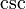$\csc$ (or sometimes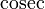$\operatorname{cosec}$) is defined as the composite of the reciprocal function and the sine function. Explicitly, it is defined as follows: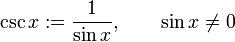$\csc x := \frac{1}{\sin x}, \qquad \sin x \ne 0$

The cosecant function is not defined at integer multiples of$\pi$, because the sine function takes the value zero at these points.

## Key data

Item Value
default domain all reals except integer multiples of$\pi$
range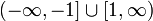$(-\infty,-1] \cup [1,\infty)$, i.e.,$\{ y \mid |y| \ge 1 \}$
no absolute minimum value or absolute maximum value.
period$2\pi$
vertical asymptotes all lines of the form$x = n\pi$,$n$ varying over integers.
For$n$ even, the left hand limit is$-\infty$ and the right hand limit is$+\infty$.
For$n$ odd, the left hand limit is$+\infty$ and the right hand limit is$-\infty$.
local minimum values and points of attainment local minimum value of 1, attained at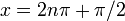$x = 2n\pi + \pi/2$,$n$ varying over integers.
local maximum values and points of attainment local maximum value of -1, attained at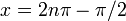$x = 2n\pi - \pi/2$,$n$ varying over integers.
points of inflection none
first derivative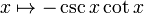$x \mapsto - \csc x \cot x$
antiderivative$x \mapsto \ln|\csc x - \cot x| + C = -\ln|\csc x + \cot x| + C$